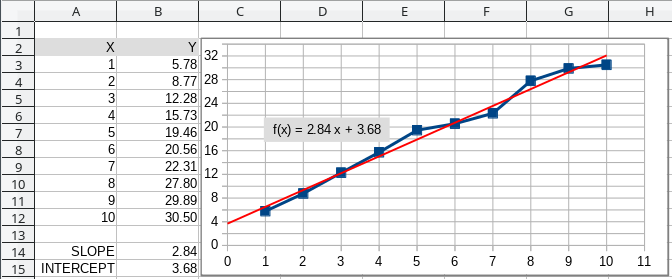# How can I reference the fit parameters of a trend line?

For making projections, I would like to extrapolate my data using the parameters from the fitted trend line in a graph/chart. At present I need to type this information into the cells every time the fit changes. Is there any way to make a reference in a cell to the fit parameters. That would be awesome!

Hello,

I’m not sure, If I got you right, but for linear trendline there is a `SLOPE()` and a `INTERCEPT()` function to get the parameters required for a linear function and with these you can perform a linear extrapolation.

In the example below

`=SLOPE(B3:B12,A3:A12)` and `=INTERCEPT(B3:B12,A3:A12)`